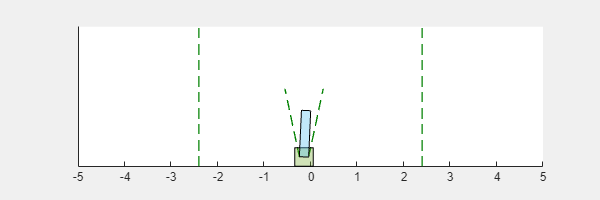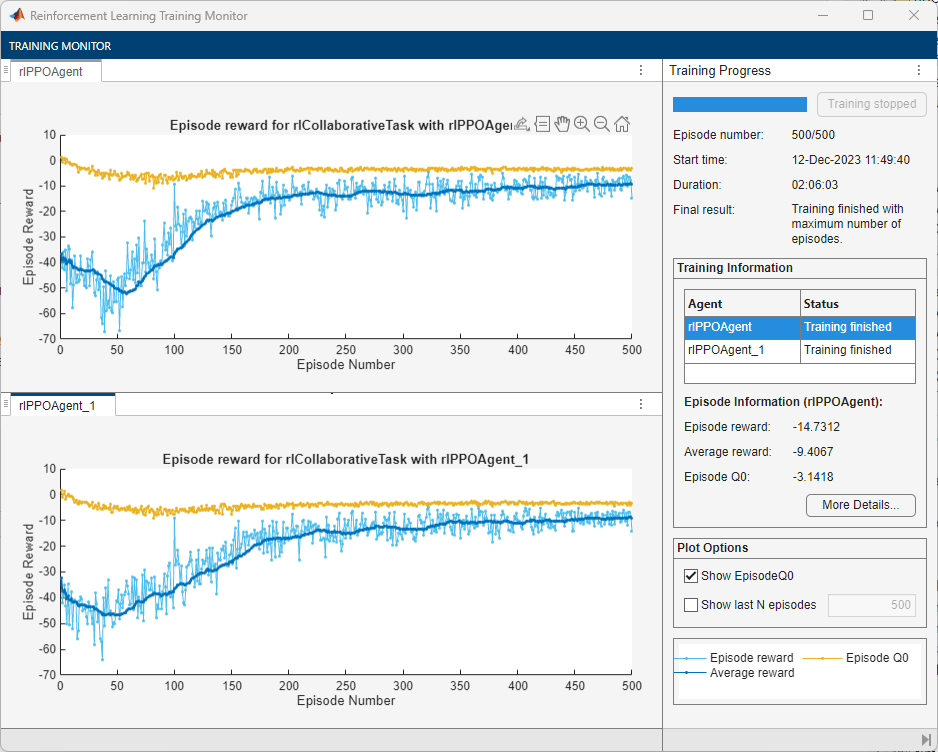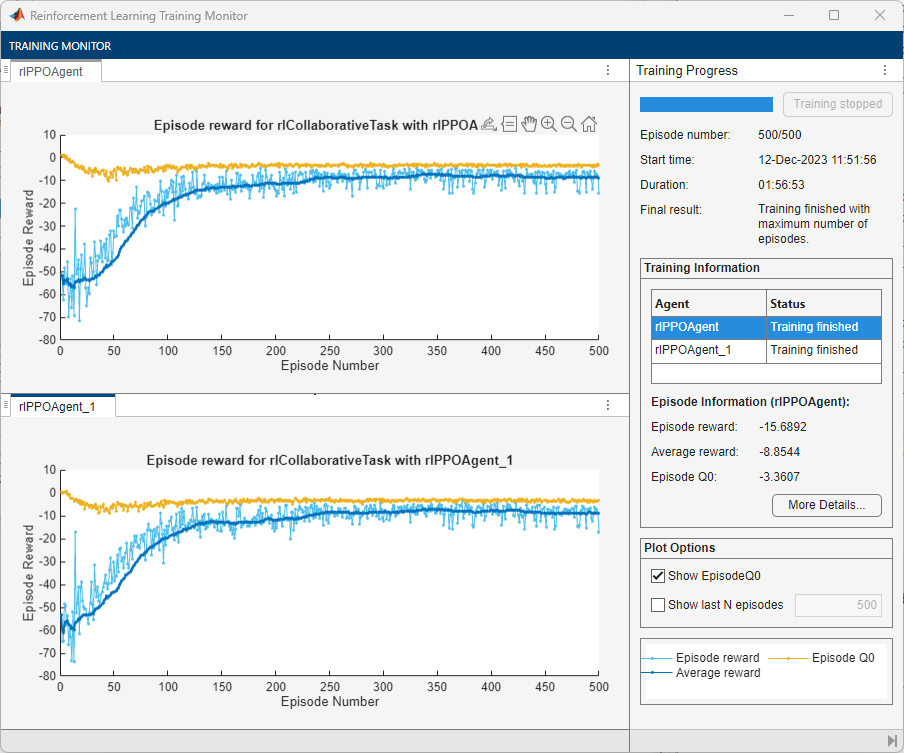# train

Train reinforcement learning agents within a specified environment

## Syntax

``trainStats = train(env,agents)``
``trainStats = train(agents,env)``
``env = train(___,trainOpts)``

## Description

````trainStats = train(env,agents)` trains one or more reinforcement learning agents within a specified environment, using default training options. Although `agents` is an input argument, after each training episode, `train` updates the parameters of each agent specified in `agents` to maximize their expected long-term reward from the environment. When training terminates, `agents` reflects the state of each agent at the end of the final training episode.```
````trainStats = train(agents,env)` performs the same training as the previous syntax.```

example

````env = train(___,trainOpts)` trains `agents` within `env`, using the training options object `trainOpts`. Use training options to specify training parameters such as the criteria for terminating training, when to save agents, the maximum number of episodes to train, and the maximum number of steps per episode. Use this syntax after any of the input arguments in the previous syntaxes.```

## Examples

collapse all

Train the agent configured in the Train PG Agent to Balance Cart-Pole System example, within the corresponding environment. The observation from the environment is a vector containing the position and velocity of a cart, as well as the angular position and velocity of the pole. The action is a scalar with two possible elements (a force of either -10 or 10 Newtons applied to a cart).

Load the file containing the environment and a PG agent already configured for it.

`load RLTrainExample.mat`

Specify some training parameters using `rlTrainingOptions`. These parameters include the maximum number of episodes to train, the maximum steps per episode, and the conditions for terminating training. For this example, use a maximum of 1000 episodes and 500 steps per episode. Instruct the training to stop when the average reward over the previous five episodes reaches 500. Create a default options set and use dot notation to change some of the parameter values.

```trainOpts = rlTrainingOptions; trainOpts.MaxEpisodes = 1000; trainOpts.MaxStepsPerEpisode = 500; trainOpts.StopTrainingCriteria = "AverageReward"; trainOpts.StopTrainingValue = 500; trainOpts.ScoreAveragingWindowLength = 5;```

During training, the `train` command can save candidate agents that give good results. Further configure the training options to save an agent when the episode reward exceeds 500. Save the agent to a folder called `savedAgents`.

```trainOpts.SaveAgentCriteria = "EpisodeReward"; trainOpts.SaveAgentValue = 500; trainOpts.SaveAgentDirectory = "savedAgents";```

Finally, turn off the command-line display. Turn on the Reinforcement Learning Episode Manager so you can observe the training progress visually.

```trainOpts.Verbose = false; trainOpts.Plots = "training-progress";```

You are now ready to train the PG agent. For the predefined cart-pole environment used in this example, you can use `plot` to generate a visualization of the cart-pole system.

`plot(env)`When you run this example, both this visualization and the Reinforcement Learning Episode Manager update with each training episode. Place them side by side on your screen to observe the progress, and train the agent. (This computation can take 20 minutes or more.)

`trainingInfo = train(agent,env,trainOpts);`Episode Manager shows that the training successfully reaches the termination condition of a reward of 500 averaged over the previous five episodes. At each training episode, `train` updates `agent` with the parameters learned in the previous episode. When training terminates, you can simulate the environment with the trained agent to evaluate its performance. The environment plot updates during simulation as it did during training.

```simOptions = rlSimulationOptions('MaxSteps',500); experience = sim(env,agent,simOptions);```During training, `train` saves to disk any agents that meet the condition specified with `trainOps.SaveAgentCritera` and `trainOpts.SaveAgentValue`. To test the performance of any of those agents, you can load the data from the data files in the folder you specified using `trainOpts.SaveAgentDirectory`, and simulate the environment with that agent.

This example shows how to set up a multi-agent training session on a Simulink® environment. In the example, you train two agents to collaboratively perform the task of moving an object.The environment in this example is a frictionless two dimensional surface containing elements represented by circles. A target object C is represented by the blue circle with a radius of 2 m, and robots A (red) and B (green) are represented by smaller circles with radii of 1 m each. The robots attempt to move object C outside a circular ring of a radius 8 m by applying forces through collision. All elements within the environment have mass and obey Newton's laws of motion. In addition, contact forces between the elements and the environment boundaries are modeled as spring and mass damper systems. The elements can move on the surface through the application of externally applied forces in the X and Y directions. There is no motion in the third dimension and the total energy of the system is conserved.

Create the set of parameters required for this example.

`rlCollaborativeTaskParams`

```mdl = "rlCollaborativeTask"; open_system(mdl)```For this environment:

• The 2-dimensional space is bounded from –12 m to 12 m in both the X and Y directions.

• The contact spring stiffness and damping values are 100 N/m and 0.1 N/m/s, respectively.

• The agents share the same observations for positions, velocities of A, B, and C and the action values from the last time step.

• The simulation terminates when object C moves outside the circular ring.

• At each time step, the agents receive the following reward:

`$\begin{array}{l}{\mathit{r}}_{\mathit{A}}={\mathit{r}}_{\mathrm{global}}+{\mathit{r}}_{\mathrm{local},\mathit{A}}\\ {\mathit{r}}_{\mathit{B}}={\mathit{r}}_{\mathrm{global}}+{\mathit{r}}_{\mathrm{local},\mathit{B}}\\ {\mathit{r}}_{\mathrm{global}}=0.001{\mathit{d}}_{\mathit{c}}\\ {\mathit{r}}_{\mathrm{local},\mathit{A}}=-0.005{\mathit{d}}_{\mathrm{AC}}-0.008{\mathit{u}}_{\mathit{A}}^{2}\\ {\mathit{r}}_{\mathrm{local},\mathit{B}}=-0.005{\mathit{d}}_{\mathrm{BC}}-0.008{\mathit{u}}_{\mathit{B}}^{2}\end{array}$`

Here:

• ${\mathit{r}}_{\mathit{A}}$and ${\mathit{r}}_{\mathit{B}}$ are the rewards received by agents A and B, respectively.

• ${\mathit{r}}_{\mathrm{global}}$ is a team reward that is received by both agents as object C moves closer towards the boundary of the ring.

• ${\mathit{r}}_{\mathrm{local},\mathit{A}}$ and ${\mathit{r}}_{\mathrm{local},\mathit{B}}$ are local penalties received by agents A and B based on their distances from object C and the magnitude of the action from the last time step.

• ${\mathit{d}}_{\mathit{C}}$ is the distance of object C from the center of the ring.

• ${\mathit{d}}_{\mathrm{AC}}$ and ${\mathit{d}}_{\mathrm{BC}}$ are the distances between agent A and object C and agent B and object C, respectively.

• ${\mathit{u}}_{\mathit{A}}$ and ${\mathit{u}}_{\mathit{B}}$ are the action values of agents A and B from the last time step.

This example uses proximal policy optimization (PPO) agents with discrete action spaces. To learn more about PPO agents, see Proximal Policy Optimization Agents. The agents apply external forces on the robots that result in motion. At every time step, the agents select the actions ${\mathit{u}}_{\mathit{A},\mathit{B}}=\left[{\mathit{F}}_{\mathit{X}}\text{\hspace{0.17em}},{\mathit{F}}_{\mathit{Y}}\right]$, where ${\mathit{F}}_{\mathit{X}},{\mathit{F}}_{\mathit{Y}}$ is one of the following pairs of externally applied forces.

`${\mathit{F}}_{\mathit{X}}=-1.0\text{\hspace{0.17em}}\mathit{N},\text{\hspace{0.17em}\hspace{0.17em}}{\mathit{F}}_{\mathit{Y}}=-1.0\text{\hspace{0.17em}}\mathit{N}$`

`${\mathit{F}}_{\mathit{X}}=-1.0\text{\hspace{0.17em}}\mathit{N},\text{\hspace{0.17em}\hspace{0.17em}}{\mathit{F}}_{\mathit{Y}}=0$`

`${\mathit{F}}_{\mathit{X}}=-1.0\text{\hspace{0.17em}}\mathit{N},\text{\hspace{0.17em}\hspace{0.17em}}{\mathit{F}}_{\mathit{Y}}=1.0\text{\hspace{0.17em}}\mathit{N}$`

`${\mathit{F}}_{\mathit{X}}=0,\text{\hspace{0.17em}\hspace{0.17em}\hspace{0.17em}\hspace{0.17em}\hspace{0.17em}\hspace{0.17em}\hspace{0.17em}\hspace{0.17em}\hspace{0.17em}\hspace{0.17em}\hspace{0.17em}\hspace{0.17em}\hspace{0.17em}\hspace{0.17em}\hspace{0.17em}}{\mathit{F}}_{\mathit{Y}}=-1.0\text{\hspace{0.17em}}\mathit{N}$`

`${\mathit{F}}_{\mathit{X}}=0,\text{\hspace{0.17em}\hspace{0.17em}\hspace{0.17em}\hspace{0.17em}\hspace{0.17em}\hspace{0.17em}\hspace{0.17em}\hspace{0.17em}\hspace{0.17em}\hspace{0.17em}\hspace{0.17em}\hspace{0.17em}\hspace{0.17em}\hspace{0.17em}\hspace{0.17em}}{\mathit{F}}_{\mathit{Y}}=0$`

`${\mathit{F}}_{\mathit{X}}=0,\text{\hspace{0.17em}\hspace{0.17em}\hspace{0.17em}\hspace{0.17em}\hspace{0.17em}\hspace{0.17em}\hspace{0.17em}\hspace{0.17em}\hspace{0.17em}\hspace{0.17em}\hspace{0.17em}\hspace{0.17em}\hspace{0.17em}\hspace{0.17em}\hspace{0.17em}}{\mathit{F}}_{\mathit{Y}}=1.0\text{\hspace{0.17em}}\mathit{N}$`

`${\mathit{F}}_{\mathit{X}}=1.0\text{\hspace{0.17em}}\mathit{N},\text{\hspace{0.17em}\hspace{0.17em}\hspace{0.17em}\hspace{0.17em}\hspace{0.17em}}{\mathit{F}}_{\mathit{Y}}=-1.0\text{\hspace{0.17em}}\mathit{N}$`

`${\mathit{F}}_{\mathit{X}}=1.0\text{\hspace{0.17em}}\mathit{N},\text{\hspace{0.17em}\hspace{0.17em}}\text{\hspace{0.17em}\hspace{0.17em}\hspace{0.17em}}{\mathit{F}}_{\mathit{Y}}=0$`

`${\mathit{F}}_{\mathit{X}}=1.0\text{\hspace{0.17em}}\mathit{N},\text{\hspace{0.17em}\hspace{0.17em}\hspace{0.17em}\hspace{0.17em}\hspace{0.17em}}{\mathit{F}}_{\mathit{Y}}=1.0\text{\hspace{0.17em}}\mathit{N}$`

Create Environment

To create a multi-agent environment, specify the block paths of the agents using a string array. Also, specify the observation and action specification objects using cell arrays. The order of the specification objects in the cell array must match the order specified in the block path array. When agents are available in the MATLAB workspace at the time of environment creation, the observation and action specification arrays are optional. For more information on creating multi-agent environments, see `rlSimulinkEnv`.

Create the I/O specifications for the environment. In this example, the agents are homogeneous and have the same I/O specifications.

```% Number of observations numObs = 16; % Number of actions numAct = 2; % Maximum value of externally applied force (N) maxF = 1.0; % I/O specifications for each agent oinfo = rlNumericSpec([numObs,1]); ainfo = rlFiniteSetSpec({ [-maxF -maxF] [-maxF 0 ] [-maxF maxF] [ 0 -maxF] [ 0 0 ] [ 0 maxF] [ maxF -maxF] [ maxF 0 ] [ maxF maxF]}); oinfo.Name = 'observations'; ainfo.Name = 'forces';```

```blks = ["rlCollaborativeTask/Agent A", "rlCollaborativeTask/Agent B"]; obsInfos = {oinfo,oinfo}; actInfos = {ainfo,ainfo}; env = rlSimulinkEnv(mdl,blks,obsInfos,actInfos);```

Specify a reset function for the environment. The reset function `resetRobots` ensures that the robots start from random initial positions at the beginning of each episode.

`env.ResetFcn = @(in) resetRobots(in,RA,RB,RC,boundaryR);`

Create Agents

PPO agents rely on actor and critic representations to learn the optimal policy. In this example, the agents maintain neural network-based function approximators for the actor and critic.

Create the critic neural network and representation. The output of the critic network is the state value function $\mathit{V}\left(\mathit{s}\right)$ for state $\mathit{s}$.

```% Reset the random seed to improve reproducibility rng(0) % Critic networks criticNetwork = [... featureInputLayer(oinfo.Dimension(1),'Normalization','none','Name','observation') fullyConnectedLayer(128,'Name','CriticFC1','WeightsInitializer','he') reluLayer('Name','CriticRelu1') fullyConnectedLayer(64,'Name','CriticFC2','WeightsInitializer','he') reluLayer('Name','CriticRelu2') fullyConnectedLayer(32,'Name','CriticFC3','WeightsInitializer','he') reluLayer('Name','CriticRelu3') fullyConnectedLayer(1,'Name','CriticOutput')]; % Critic representations criticOpts = rlRepresentationOptions('LearnRate',1e-4); criticA = rlValueRepresentation(criticNetwork,oinfo,'Observation',{'observation'},criticOpts); criticB = rlValueRepresentation(criticNetwork,oinfo,'Observation',{'observation'},criticOpts);```

The outputs of the actor network are the probabilities $\pi \left(\mathit{a}|\mathit{s}\right)$of taking each possible action pair at a certain state $\mathit{s}$. Create the actor neural network and representation.

```% Actor networks actorNetwork = [... featureInputLayer(oinfo.Dimension(1),'Normalization','none','Name','observation') fullyConnectedLayer(128,'Name','ActorFC1','WeightsInitializer','he') reluLayer('Name','ActorRelu1') fullyConnectedLayer(64,'Name','ActorFC2','WeightsInitializer','he') reluLayer('Name','ActorRelu2') fullyConnectedLayer(32,'Name','ActorFC3','WeightsInitializer','he') reluLayer('Name','ActorRelu3') fullyConnectedLayer(numel(ainfo.Elements),'Name','Action') softmaxLayer('Name','SM')]; % Actor representations actorOpts = rlRepresentationOptions('LearnRate',1e-4); actorA = rlStochasticActorRepresentation(actorNetwork,oinfo,ainfo,... 'Observation',{'observation'},actorOpts); actorB = rlStochasticActorRepresentation(actorNetwork,oinfo,ainfo,... 'Observation',{'observation'},actorOpts);```

Create the agents. Both agents use the same options.

```agentOptions = rlPPOAgentOptions(... 'ExperienceHorizon',256,... 'ClipFactor',0.125,... 'EntropyLossWeight',0.001,... 'MiniBatchSize',64,... 'NumEpoch',3,... 'AdvantageEstimateMethod','gae',... 'GAEFactor',0.95,... 'SampleTime',Ts,... 'DiscountFactor',0.9995); agentA = rlPPOAgent(actorA,criticA,agentOptions); agentB = rlPPOAgent(actorB,criticB,agentOptions);```

During training, agents collect experiences until either the experience horizon of 256 steps or the episode termination is reached, and then train from mini-batches of 64 experiences. This example uses an objective function clip factor of 0.125 to improve training stability and a discount factor of 0.9995 to encourage long-term rewards.

Train Agents

Specify the following training options to train the agents.

• Run the training for at most 1000 episodes, with each episode lasting at most 5000 time steps.

• Stop the training of an agent when its average reward over 100 consecutive episodes is –10 or more.

```maxEpisodes = 1000; maxSteps = 5e3; trainOpts = rlTrainingOptions(... 'MaxEpisodes',maxEpisodes,... 'MaxStepsPerEpisode',maxSteps,... 'ScoreAveragingWindowLength',100,... 'Plots','training-progress',... 'StopTrainingCriteria','AverageReward',... 'StopTrainingValue',-10);```

To train multiple agents, specify an array of agents to the `train` function. The order of agents in the array must match the order of agent block paths specified during environment creation. Doing so ensures that the agent objects are linked to their appropriate I/O interfaces in the environment. Training these agents can take several hours to complete, depending on the available computational power.

The MAT file `rlCollaborativeTaskAgents` contains a set of pretrained agents. You can load the file and to view the performance of the agents. To train the agents yourself, set `doTraining` to `true`.

```doTraining = false; if doTraining stats = train([agentA, agentB],env,trainOpts); else load('rlCollaborativeTaskAgents.mat'); end```

The following figure shows a snapshot of training progress. You can expect different results due to randomness in the training process.Simulate Agents

Simulate the trained agents within the environment.

```simOptions = rlSimulationOptions('MaxSteps',maxSteps); exp = sim(env,[agentA agentB],simOptions);```For more information on agent simulation, see `rlSimulationOptions` and `sim`.

## Input Arguments

collapse all

Agents to train, specified as a reinforcement learning agent object, such as `rlACAgent` or `rlDDPGAgent`, or as an array of such objects.

If `env` is a multi-agent environment created with `rlSimulinkEnv`, specify agents as an array. The order of the agents in the array must match the agent order used to create `env`. Multi-agent simulation is not supported for MATLAB® environments.

Note

`train` updates `agents` at each training episode. When training terminates, `agents` reflects the state of each agent at the end of the final training episode. Therefore, the rewards obtained by the final agents are not necessarily the highest achieved during the training process, due to continuous exploration. To save agents during training, create an `rlTrainingOptions` object specifying the SaveAgentCriteria and SaveAgentValue properties and pass it to `train` as a `trainOpts` argument.

For more information about how to create and configure agents for reinforcement learning, see Reinforcement Learning Agents.

Environment in which the agents act, specified as one of the following kinds of reinforcement learning environment object:

When `env` is a Simulink environment, calling `train` compiles and simulates the model associated with the environment.

Training parameters and options, specified as an `rlTrainingOptions` object. Use this argument to specify such parameters and options as:

• Criteria for ending training

• Criteria for saving candidate agents

• How to display training progress

• Options for parallel computing

For details, see `rlTrainingOptions`.

## Output Arguments

collapse all

Training episode data, returned as a structure containing the following fields.

Episode numbers, returned as the column vector `[1;2;…;N]`, where `N` is the number of episodes in the training run. This vector is useful if you want to plot the evolution of other quantities from episode to episode.

Reward for each episode, returned in a column vector of length `N`. Each entry contains the reward for the corresponding episode.

Number of steps in each episode, returned in a column vector of length `N`. Each entry contains the number of steps in the corresponding episode.

Average reward over the averaging window specified in `trainOpts`, returned as a column vector of length `N`. Each entry contains the average award computed at the end of the corresponding episode.

Total number of agent steps in training, returned as a column vector of length `N`. Each entry contains the cumulative sum of the entries in `EpisodeSteps` up to that point.

Critic estimate of long-term reward using the current agent and the environment initial conditions, returned as a column vector of length `N`. Each entry is the critic estimate (Q0) for the agent of the corresponding episode. This field is present only for agents that have critics, such as `rlDDPGAgent` and `rlDQNAgent`.

Information collected during the simulations performed for training, returned as:

• For training in MATLAB environments, a structure containing the field `SimulationError`. This field is a column vector with one entry per episode. When the `StopOnError` option of `rlTrainingOptions` is `"off"`, each entry contains any errors that occurred during the corresponding episode.

• For training in Simulink environments, a vector of `Simulink.SimulationOutput` objects containing simulation data recorded during the corresponding episode. Recorded data for an episode includes any signals and states that the model is configured to log, simulation metadata, and any errors that occurred during the corresponding episode.

## Tips

• `train` updates the agents as training progresses. To preserve the original agent parameters for later use, save the agents to a MAT-file.

• By default, calling `train` opens the Reinforcement Learning Episode Manager, which lets you visualize the progress of the training. The Episode Manager plot shows the reward for each episode, a running average reward value, and the critic estimate Q0 (for agents that have critics). The Episode Manager also displays various episode and training statistics. To turn off the Reinforcement Learning Episode Manager, set the `Plots` option of `trainOpts` to `"none"`.

• If you use a predefined environment for which there is a visualization, you can use `plot(env)` to visualize the environment. If you call `plot(env)` before training, then the visualization updates during training to allow you to visualize the progress of each episode. (For custom environments, you must implement your own `plot` method.)

• Training terminates when the conditions specified in `trainOpts` are satisfied. To terminate training in progress, in the Reinforcement Learning Episode Manager, click Stop Training. Because `train` updates the agent at each episode, you can resume training by calling `train(agent,env,trainOpts)` again, without losing the trained parameters learned during the first call to `train`.

• During training, you can save candidate agents that meet conditions you specify with `trainOpts`. For instance, you can save any agent whose episode reward exceeds a certain value, even if the overall condition for terminating training is not yet satisfied. `train` stores saved agents in a MAT-file in the folder you specify with `trainOpts`. Saved agents can be useful, for instance, to allow you to test candidate agents generated during a long-running training process. For details about saving criteria and saving location, see `rlTrainingOptions`.

## Algorithms

In general, `train` performs the following iterative steps:

1. Initialize `agent`.

2. For each episode:

1. Reset the environment.

2. Get the initial observation s0 from the environment.

3. Compute the initial action a0 = μ(s0).

4. Set the current action to the initial action (aa0) and set the current observation to the initial observation (ss0).

5. While the episode is not finished or terminated:

1. Step the environment with action a to obtain the next observation s' and the reward r.

2. Learn from the experience set (s,a,r,s').

3. Compute the next action a' = μ(s').

4. Update the current action with the next action (aa') and update the current observation with the next observation (ss').

5. Break if the episode termination conditions defined in the environment are met.

3. If the training termination condition defined by `trainOpts` is met, terminate training. Otherwise, begin the next episode.

The specifics of how `train` performs these computations depends on your configuration of the agent and environment. For instance, resetting the environment at the start of each episode can include randomizing initial state values, if you configure your environment to do so.